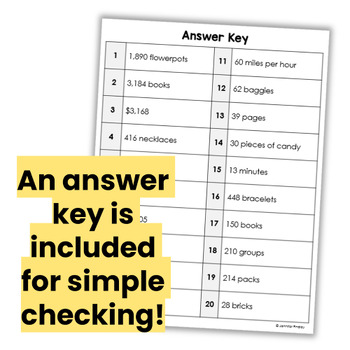# 4th Grade Multiplication and Division Word Problems (Interactive Notebook)Subject
Resource Type
File Type
PDF (1 MB|20 pages)
Standards
\$2.40
List Price:
\$3.00
You Save:
\$0.60
\$2.40
List Price:
\$3.00
You Save:
\$0.60
Also included in:
1. Do you need word problems for your students to complete to practice all of the common core standards and then some? This discounted bundle of Interactive Notebook Word Problems will have you set for the entire year.Click here to see the 5th grade version.This bundle includes a total of 146 word prob
\$24.00
\$11.20
Save \$12.80
• Product Description
• Standards

This resource includes 20 multiplication and division word problems for use in interactive notebooks. The word problems are aligned with 4th grade math standards and include multiplying word problems (10 problems) and dividing word problems (10 problems).

Multiplication: 3-digit by 1-digit, 4-digit by 1-digit, and 2-digit by 2-digit

Division: 3-digit by 1-digit and 4 digit by 1-digit

Click here to see a discounted BUNDLE of 4th Grade Math Interactive Notebook Word Problems that includes this resource.

Word problems are huge in Common Core and students need to be prepared to not only solve them, but to show and prove their thinking. I created this resource to help my students meet this requirement.

Depending on your printer settings and the size of the notebooks you are using, you may need to print the paper at 80-90% to have it fit in your notebook of choice.

The students write the solution on the word problem paper and then show their work, explain the steps they took or prove their answer underneath the word problem on their notebook paper.

You may also like:

Interactive Math Notebook: 4th Grade Word Problems {All Common Core Standards}: This resource is similar in format but includes a word problem for each common core standard.

5th Grade Word Problems for Interactive Notebooks: BUNDLE

4th Grade Math Centers for EVERY Common Core Standard

Thanks!

Jennifer Findley

Find whole-number quotients and remainders with up to four-digit dividends and one-digit divisors, using strategies based on place value, the properties of operations, and/or the relationship between multiplication and division. Illustrate and explain the calculation by using equations, rectangular arrays, and/or area models.
Multiply a whole number of up to four digits by a one-digit whole number, and multiply two two-digit numbers, using strategies based on place value and the properties of operations. Illustrate and explain the calculation by using equations, rectangular arrays, and/or area models.
Total Pages
20 pages